Скачать презентацию UNIVERSITY OF MASSACHUSETTS Dept of Electrical Computer

e5911eb3834265360e2109b8bc01f5cb.ppt

• Количество слайдов: 14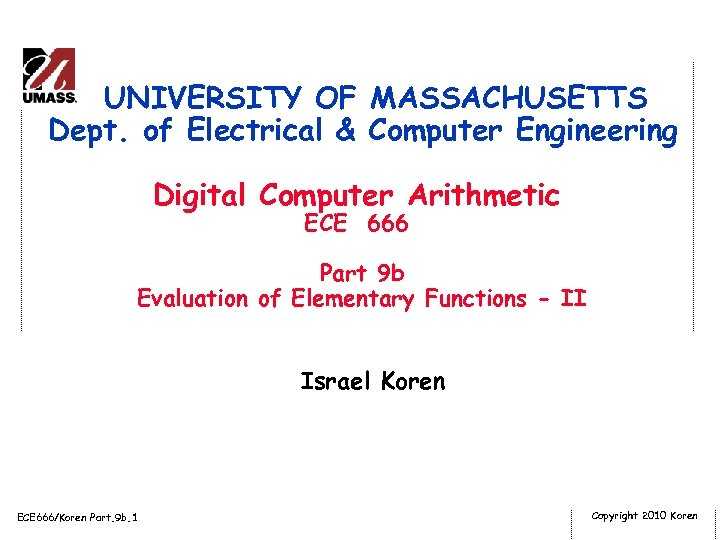UNIVERSITY OF MASSACHUSETTS Dept. of Electrical & Computer Engineering Digital Computer Arithmetic ECE 666 Part 9 b Evaluation of Elementary Functions - II Israel Koren ECE 666/Koren Part. 9 b. 1 Copyright 2010 Koren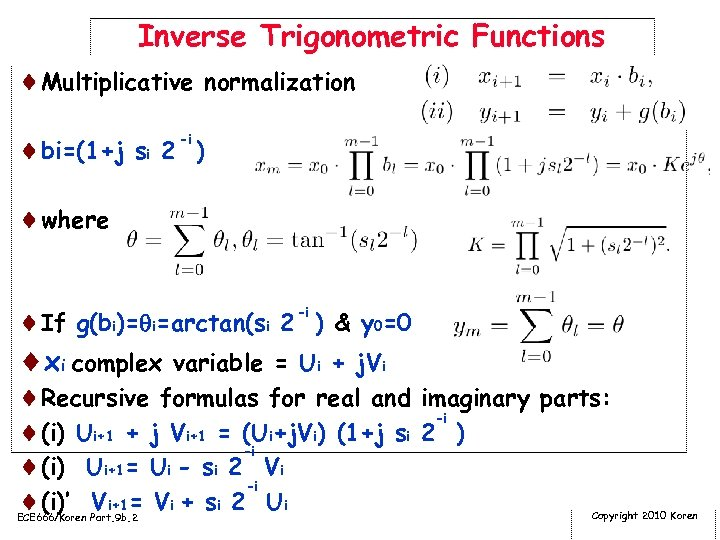Inverse Trigonometric Functions ¨Multiplicative normalization -i ¨bi=(1+j si 2 ) ¨where ¨If g(bi)= i=arctan(si 2 -i ) & y 0=0 ¨xi complex variable = Ui + j. Vi ¨Recursive formulas for real and imaginary parts: -i ¨(i) Ui+1 + j Vi+1 = (Ui+j. Vi) (1+j si 2 ) -i ¨(i) Ui+1= Ui - si 2 Vi -i ¨(i)’ Vi+1= Vi + si 2 Ui ECE 666/Koren Part. 9 b. 2 Copyright 2010 Koren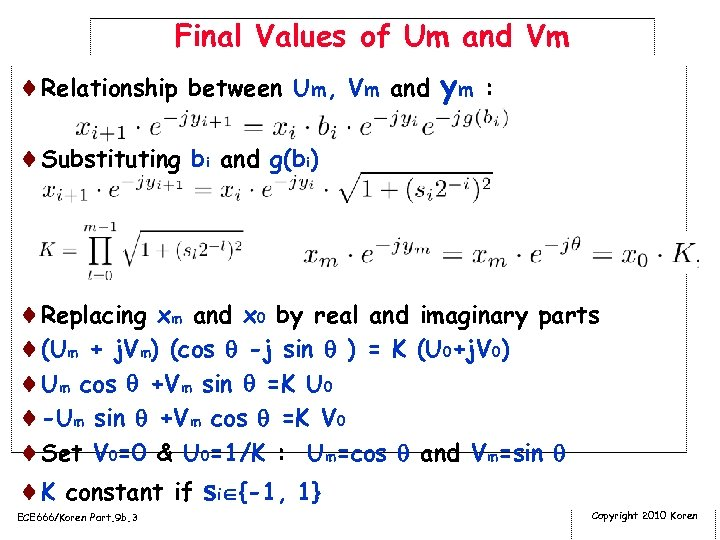Final Values of Um and Vm ¨Relationship between Um, Vm and ym : ¨Substituting bi and g(bi) ¨Replacing xm and x 0 by real and imaginary parts ¨(Um + j. Vm) (cos -j sin ) = K (U 0+j. V 0) ¨Um cos +Vm sin =K U 0 ¨-Um sin +Vm cos =K V 0 ¨Set V 0=0 & U 0=1/K : Um=cos and Vm=sin ¨K constant if si {-1, 1} ECE 666/Koren Part. 9 b. 3 Copyright 2010 Koren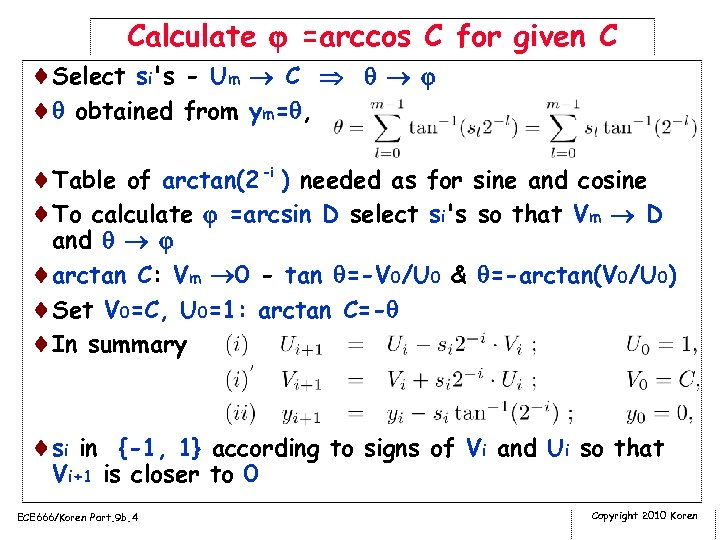Calculate =arccos C for given C ¨Select si's - Um C ¨ obtained from ym= , ¨Table of arctan(2 -i ) needed as for sine and cosine ¨To calculate =arcsin D select si's so that Vm D and ¨arctan C: Vm 0 - tan =-V 0/U 0 & =-arctan(V 0/U 0) ¨Set V 0=C, U 0=1: arctan C=- ¨In summary ¨si in {-1, 1} according to signs of Vi and Ui so that Vi+1 is closer to 0 ECE 666/Koren Part. 9 b. 4 Copyright 2010 Koren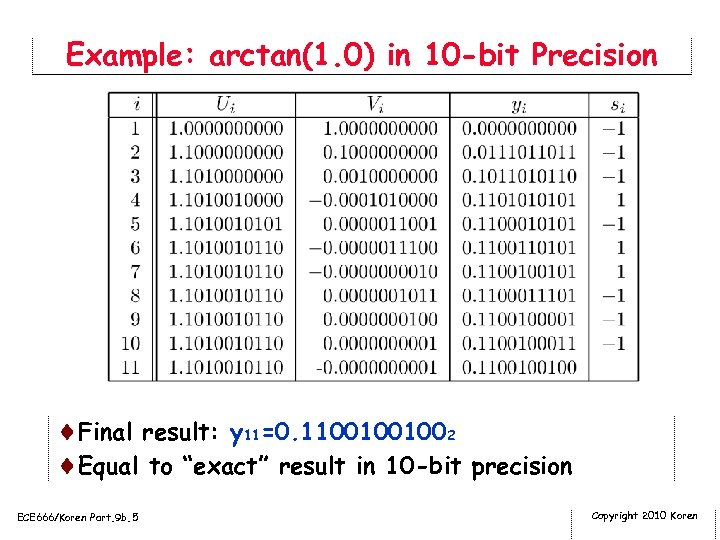Example: arctan(1. 0) in 10 -bit Precision ¨Final result: y 11=0. 11001001002 ¨Equal to “exact” result in 10 -bit precision ECE 666/Koren Part. 9 b. 5 Copyright 2010 Koren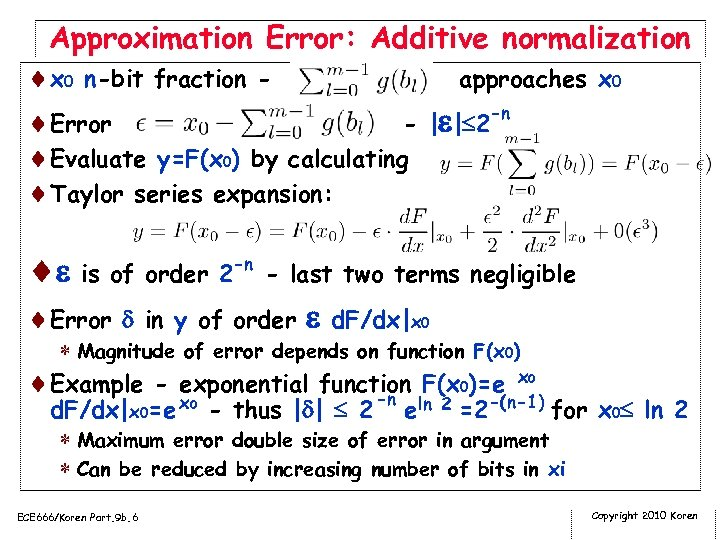Approximation Error: Additive normalization ¨x 0 n-bit fraction approaches x 0 -n ¨Error - | | 2 ¨Evaluate y=F(x 0) by calculating ¨Taylor series expansion: ¨ is of order 2 -n - last two terms negligible ¨Error in y of order d. F/dx|x 0 * Magnitude of error depends on function F(x 0) ¨Example - exponential function F(x 0)=e x 0 -n d. F/dx|x 0=e x 0 - thus | | 2 eln 2 =2 -(n-1) for x 0 ln 2 * Maximum error double size of error in argument * Can be reduced by increasing number of bits in xi ECE 666/Koren Part. 9 b. 6 Copyright 2010 Koren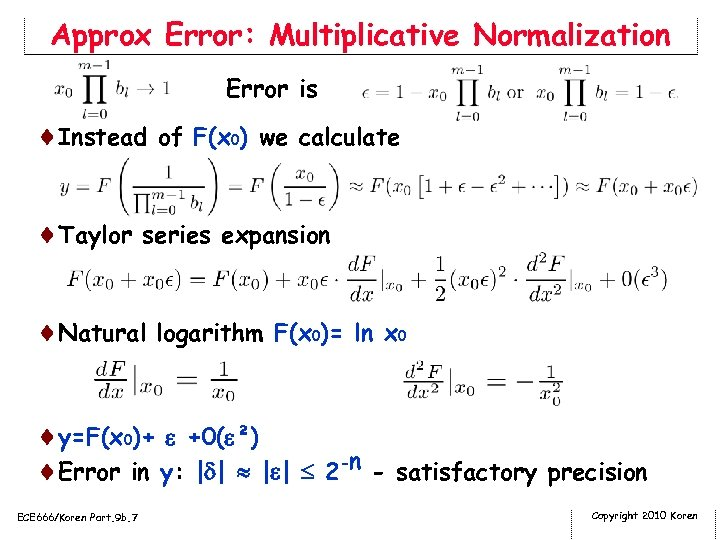Approx Error: Multiplicative Normalization Error is ¨Instead of F(x 0) we calculate ¨Taylor series expansion ¨Natural logarithm F(x 0)= ln x 0 ¨y=F(x 0)+ +0( ²) ¨Error in y: | | 2 -n - satisfactory precision ECE 666/Koren Part. 9 b. 7 Copyright 2010 Koren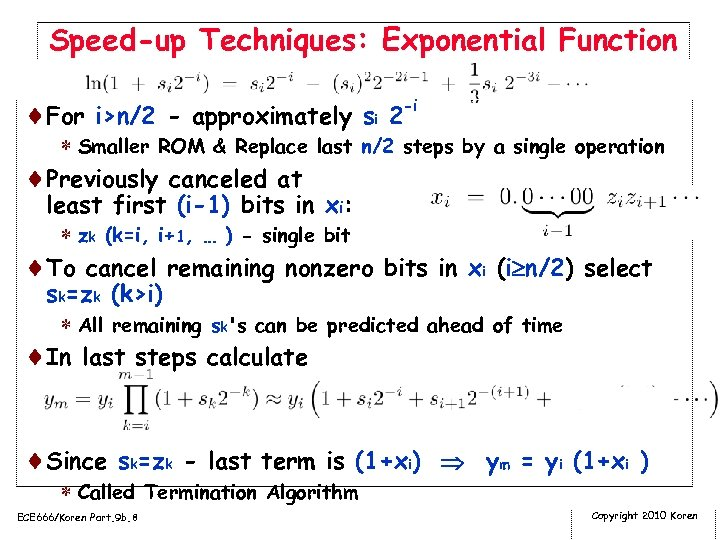Speed-up Techniques: Exponential Function ¨For i>n/2 - approximately si 2 -i * Smaller ROM & Replace last n/2 steps by a single operation ¨Previously canceled at least first (i-1) bits in xi: * zk (k=i, i+1, … ) - single bit ¨To cancel remaining nonzero bits in xi (i n/2) select sk=zk (k>i) * All remaining sk's can be predicted ahead of time ¨In last steps calculate ¨Since sk=zk - last term is (1+xi) ym = yi (1+xi ) * Called Termination Algorithm ECE 666/Koren Part. 9 b. 8 Copyright 2010 Koren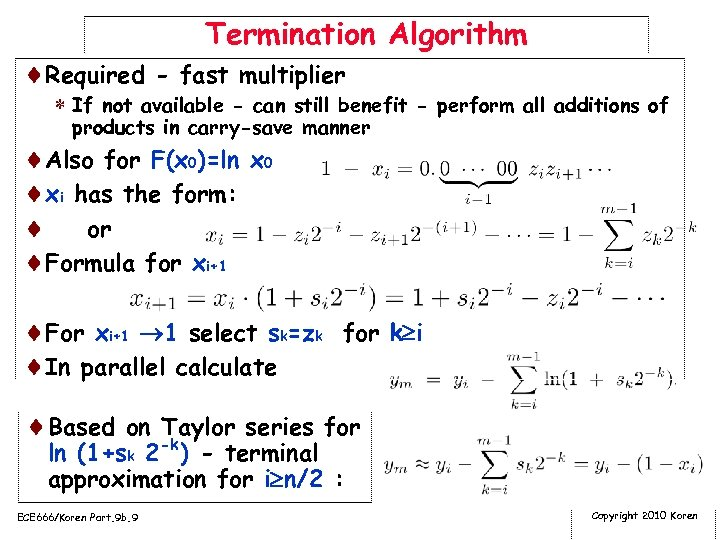Termination Algorithm ¨Required - fast multiplier * If not available - can still benefit - perform all additions of products in carry-save manner ¨Also for F(x 0)=ln x 0 ¨xi has the form: ¨ or ¨Formula for xi+1 ¨For xi+1 1 select sk=zk for k i ¨In parallel calculate ¨Based on -k Taylor series for ln (1+sk 2 ) - terminal approximation for i n/2 : ECE 666/Koren Part. 9 b. 9 Copyright 2010 Koren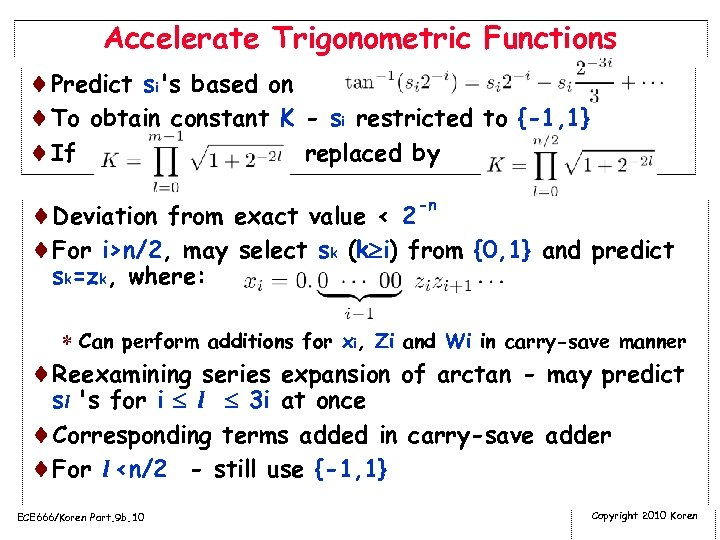Accelerate Trigonometric Functions ¨Predict si's based on ¨To obtain constant K - si restricted to {-1, 1} ¨If replaced by ¨Deviation from exact value < 2 -n ¨For i>n/2, may select sk (k i) from {0, 1} and predict sk=zk, where: * Can perform additions for xi, Zi and Wi in carry-save manner ¨Reexamining series expansion of arctan - may predict sl 's for i l 3 i at once ¨Corresponding terms added in carry-save adder ¨For l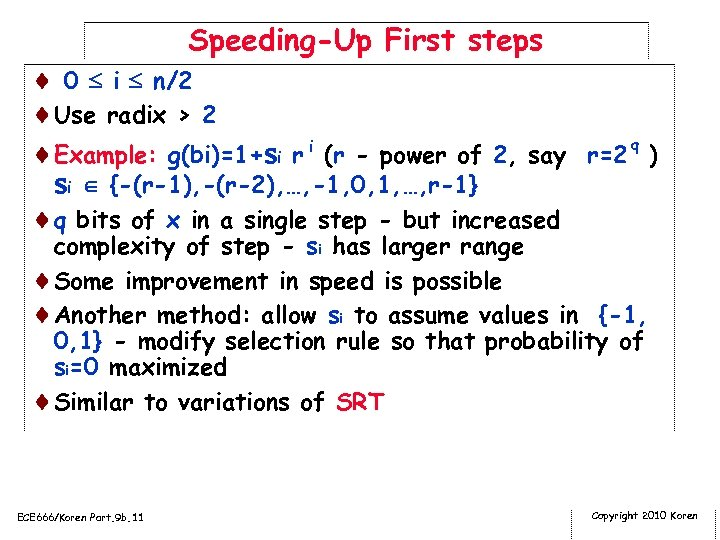Speeding-Up First steps ¨ 0 i n/2 ¨Use radix > 2 ¨Example: g(bi)=1+si r i (r - power of 2, say r=2 q ) si {-(r-1), -(r-2), …, -1, 0, 1, …, r-1} ¨q bits of x in a single step - but increased complexity of step - si has larger range ¨Some improvement in speed is possible ¨Another method: allow si to assume values in {-1, 0, 1} - modify selection rule so that probability of si=0 maximized ¨Similar to variations of SRT ECE 666/Koren Part. 9 b. 11 Copyright 2010 Koren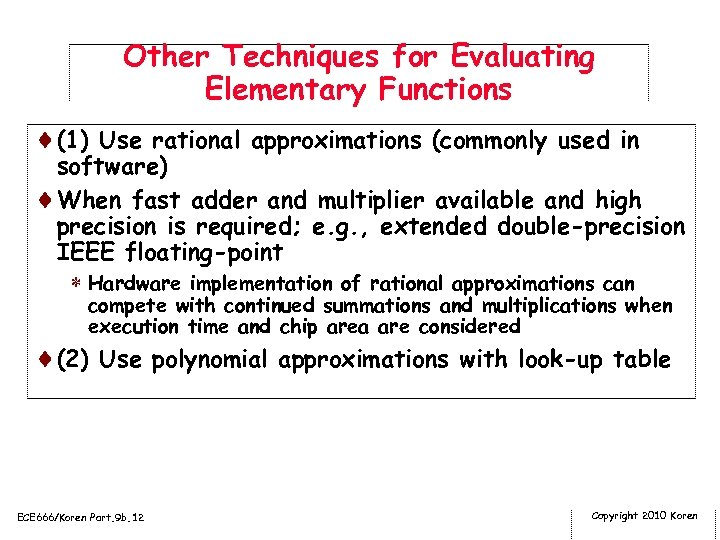Other Techniques for Evaluating Elementary Functions ¨(1) Use rational approximations (commonly used in software) ¨When fast adder and multiplier available and high precision is required; e. g. , extended double-precision IEEE floating-point * Hardware implementation of rational approximations can compete with continued summations and multiplications when execution time and chip area are considered ¨(2) Use polynomial approximations with look-up table ECE 666/Koren Part. 9 b. 12 Copyright 2010 Koren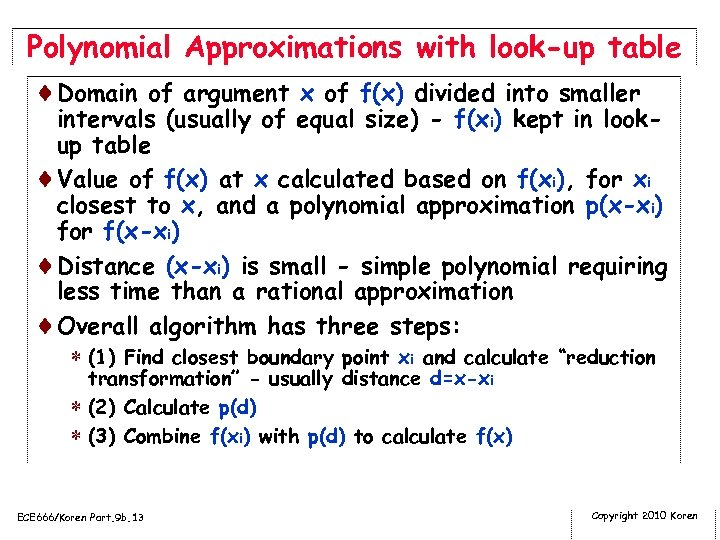Polynomial Approximations with look-up table ¨Domain of argument x of f(x) divided into smaller intervals (usually of equal size) - f(xi) kept in lookup table ¨Value of f(x) at x calculated based on f(xi), for xi closest to x, and a polynomial approximation p(x-xi) for f(x-xi) ¨Distance (x-xi) is small - simple polynomial requiring less time than a rational approximation ¨Overall algorithm has three steps: * (1) Find closest boundary point xi and calculate “reduction transformation” - usually distance d=x-xi * (2) Calculate p(d) * (3) Combine f(xi) with p(d) to calculate f(x) ECE 666/Koren Part. 9 b. 13 Copyright 2010 Koren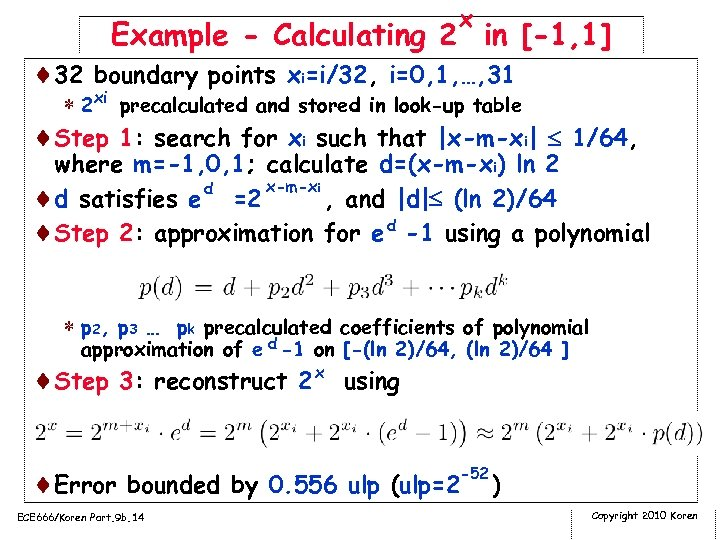x Example - Calculating 2 in [-1, 1] ¨ 32 boundary points xi=i/32, i=0, 1, …, 31 * 2 xi precalculated and stored in look-up table ¨Step 1: search for xi such that |x-m-xi| 1/64, where m=-1, 0, 1; calculate d=(x-m-xi) ln 2 x-m-xi d ¨d satisfies e =2 , and |d| (ln 2)/64 ¨Step 2: approximation for e d -1 using a polynomial * p 2, p 3 … pk precalculated coefficients of polynomial approximation of e d -1 on [-(ln 2)/64, (ln 2)/64 ] ¨Step 3: reconstruct 2 x using -52 ¨Error bounded by 0. 556 ulp (ulp=2 ECE 666/Koren Part. 9 b. 14 ) Copyright 2010 Koren